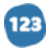# ulhii

explanatory Essay
674 words
674 wordsThe purpose of this experiment was to use measurements of change in heat and Hess’s law to compare reactions. In this lab we used the formula q=(cp)(m)(∆T). Where cp or Specific heat is the heat capacity of 1 gram of a substance in units J / (g x K). The reactions were all exothermic meaning energy was released from the system. This is the opposite of endothermic which means energy is absorbed by a system. After we had recorded the initial and final temperatures from the experiment we subtracted the final temperature from the initial temperature to find the change in temperature or (∆T). After we had obtained the change in temperature we took this number and plugged it into the formula q=(cp)(m)(∆T). This gave us the heat of the reaction, which we took and divided by the amount of mole concentration. That is how we calculated the change of heat for reaction #1. We used the same process for reactions #2 and #3, except we used the numbers we had recorded for those reactions. We then took the the change in heat from reaction #1-3 and placed them in order to find our actual heat. We then modified the setup to find the theoretical yield and compared our answers. This also proved our hypothesis because the values of the change in heat from reaction #1 and #2 should have been equal to the value of the change in heat form reaction #3. Reaction #1 and #2 were sub reactions, to reaction #3. Meaning that reaction #3 was a reaction that consisted of all the parts reaction #1 and #2 had combined. This agrees with the rules of Hess’s law which states: if a reaction is carried out in a series of steps, ∆H for the reaction will be equal to the sum of the enthalpy changes for the individual steps. For reaction #1 the data we recorded is as follow...

... middle of paper ...

...at eye level. The percent error that I ended up with was 28.07%. This means that our technique was very poor and needs improvement.
The main idea that I got out of this lab is that adding parts of heat to come up with the total is more important than it may seem. It is very useful when commercial labs are making a product. If they are producing in large quantities then it easily become a multi billion dollar project. Therefore small errors can lead to disasters. Checking individual steps can help make sure that there is nothing wrong before they have the entire product. This is something that is of many benefits to the medical field. It also helps us anticipate enthalpy changes of reactions that are too slow. For example it can help us with food cooking and pass the time it takes to rust a nail. Hess’s law is very important and is a very big contribution to society.

#### In this essay, the author

• Explains how the experiment used heat measurements and hess's law to compare reactions.
• Explains that reaction #3 was a reaction that consisted of all the parts reaction #1 and #2 had combined.
• Opines that the equipment they used was not top-of-the-line, but their measurements could have been more accurate with a little more care.

•